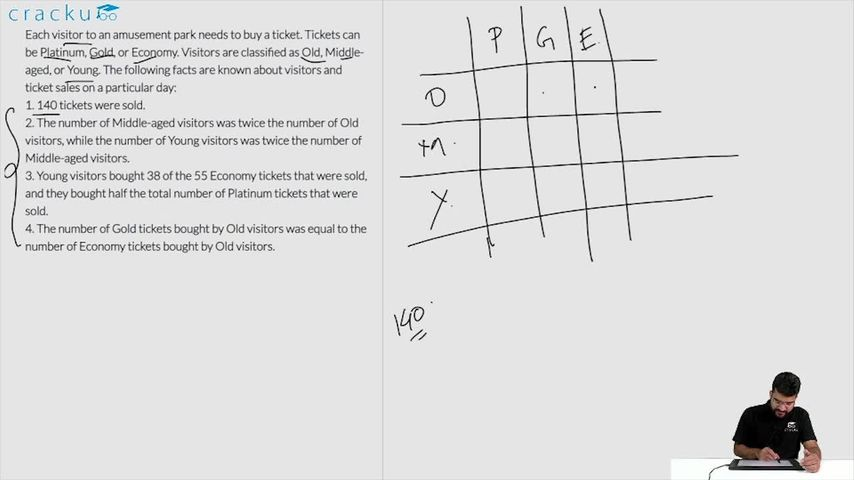### CAT 2018 Question Paper (Slot 2) Question 41

Instructions

Each visitor to an amusement park needs to buy a ticket. Tickets can be Platinum, Gold, or Economy. Visitors are classified as Old, Middle-aged, or Young. The following facts are known about visitors and ticket sales on a particular day:
1. 140 tickets were sold.
2. The number of Middle-aged visitors was twice the number of Old visitors, while the number of Young visitors was twice the number of Middle-aged visitors.
3. Young visitors bought 38 of the 55 Economy tickets that were sold, and they bought half the total number of Platinum tickets that were sold.
4. The number of Gold tickets bought by Old visitors was equal to the number of Economy tickets bought by Old visitors.

Question 41

# If the number of Old visitors buying Gold tickets was strictly greater than the number of Young visitors buying Gold tickets, then the number of Middle-aged visitors buying Gold tickets was

Solution

Let 'x' be the the number of Old visitors. Then, the number of middle-aged visitors = 2x.

Also, the number of Young visitors = 2*2x = 4x

$$\Rightarrow$$ x+2x+4x = 140

$$\Rightarrow$$ x = 20

It is given that total of 55 Economy tickets were sold out.

It is given that Young visitors half the total number of Platinum tickets that were sold.

Let 'Y' be the number of Platinum tickets bought by the Young visitors.

Then,the number of Platinum tickets sold = 2Y.

Consequently, we can say that the number of Gold tickets sold = 140 - 55 - 2Y = 85 - 2Y.

Let us assume that 'Z' is the number of Economy tickets bought by the Old visitors. It is given that the number of Gold tickets bought by Old visitors was equal to the number of Economy tickets bought by Old visitors.

It is given that the number of Old visitors buying Gold tickets was strictly greater than the number of Young visitors buying Gold tickets.

Z > 42 - Y

$$\Rightarrow$$ Z + Y > 42 ... (1)

The number of Middle-aged visitors buying Gold tickets = 43 - (Y+Z)

Since (Y+Z) > 42, then We can say that (Y+Z)$$_{min}$$ = 43.

Hence, the number of Middle-aged visitors buying Gold tickets = 43 - 43 = 0

### View Video Solution